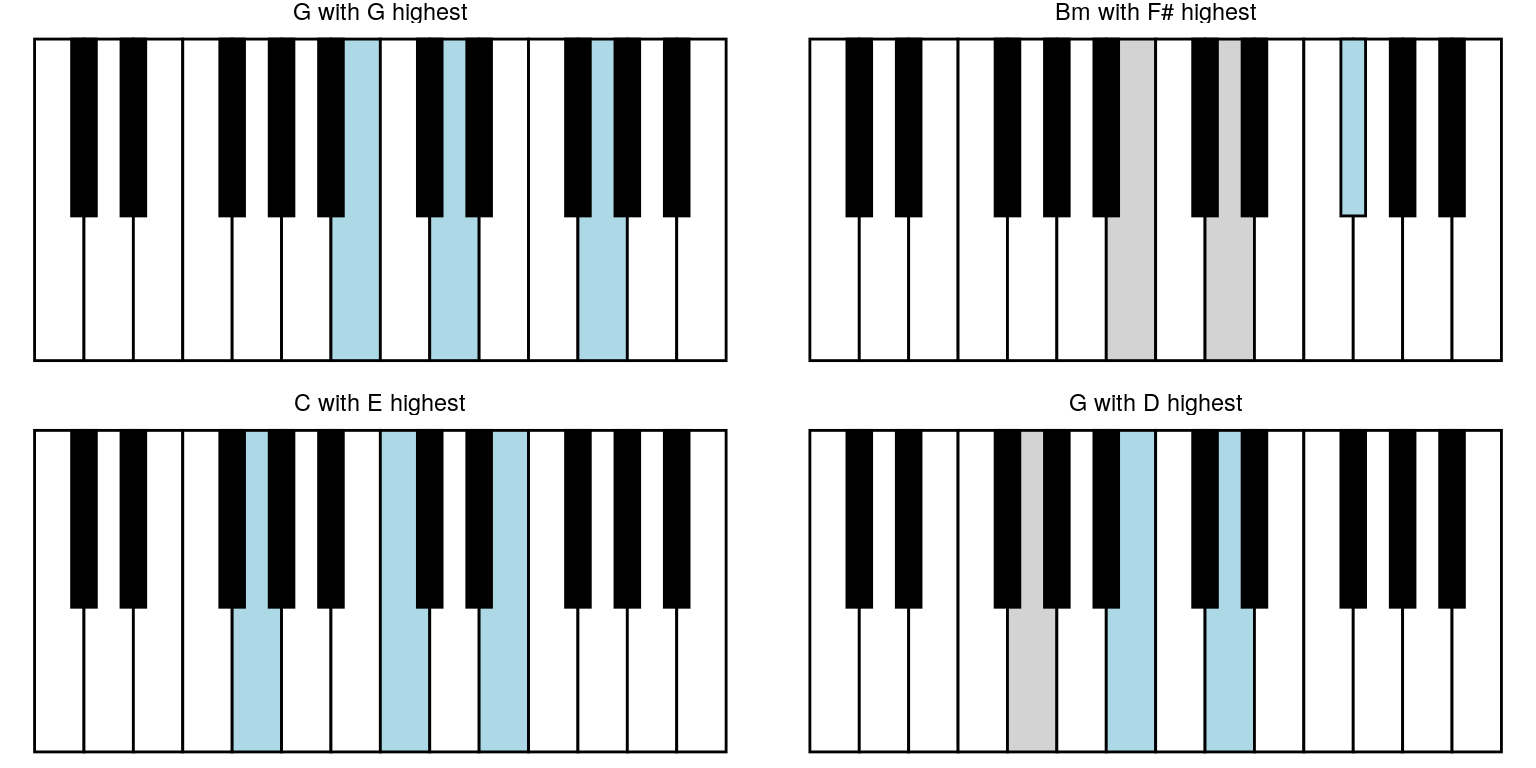library(ggplot2)
library(forcats)
library(dplyr)
library(pichor)

# Minimal example

keys_chords %>%
highlight_key_sequence(key_sequence = list(c(1, 2, 3), c(2, 3, 4))) %>%
ggpiano() +
facet_wrap(~ seq_name)
#> NULL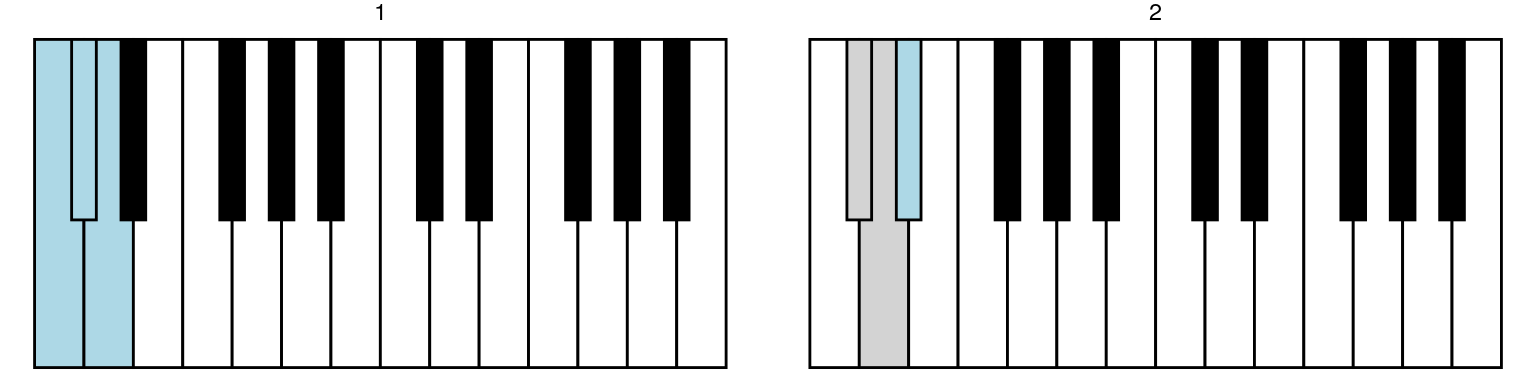# Slightly larger example

A version of “Let it be” by Beatles initially has chords G, D, Em, C, G, D, C.

chords <- list(construct_chord_major("G"),
construct_chord_major("D"),
construct_chord_minor("E"), # Em
construct_chord_major("C"),
construct_chord_major("G"),
construct_chord_major("D"),
construct_chord_major("C"))
chords
#> []
#> G chord (major) with tones G, B, D
#>
#> []
#> D chord (major) with tones D, F#/Gb, A
#>
#> []
#> Em chord (minor) with tones E, G, B
#>
#> []
#> C chord (major) with tones C, E, G
#>
#> []
#> G chord (major) with tones G, B, D
#>
#> []
#> D chord (major) with tones D, F#/Gb, A
#>
#> []
#> C chord (major) with tones C, E, G
chord_names <- sapply(chords, as.character, brief = TRUE)
key_seq <- lapply(chords, get_keys)
keys_chords %>%
highlight_key_sequence(key_sequence = key_seq) %>%
ggpiano() +
facet_wrap(~ seq_name)
#> NULL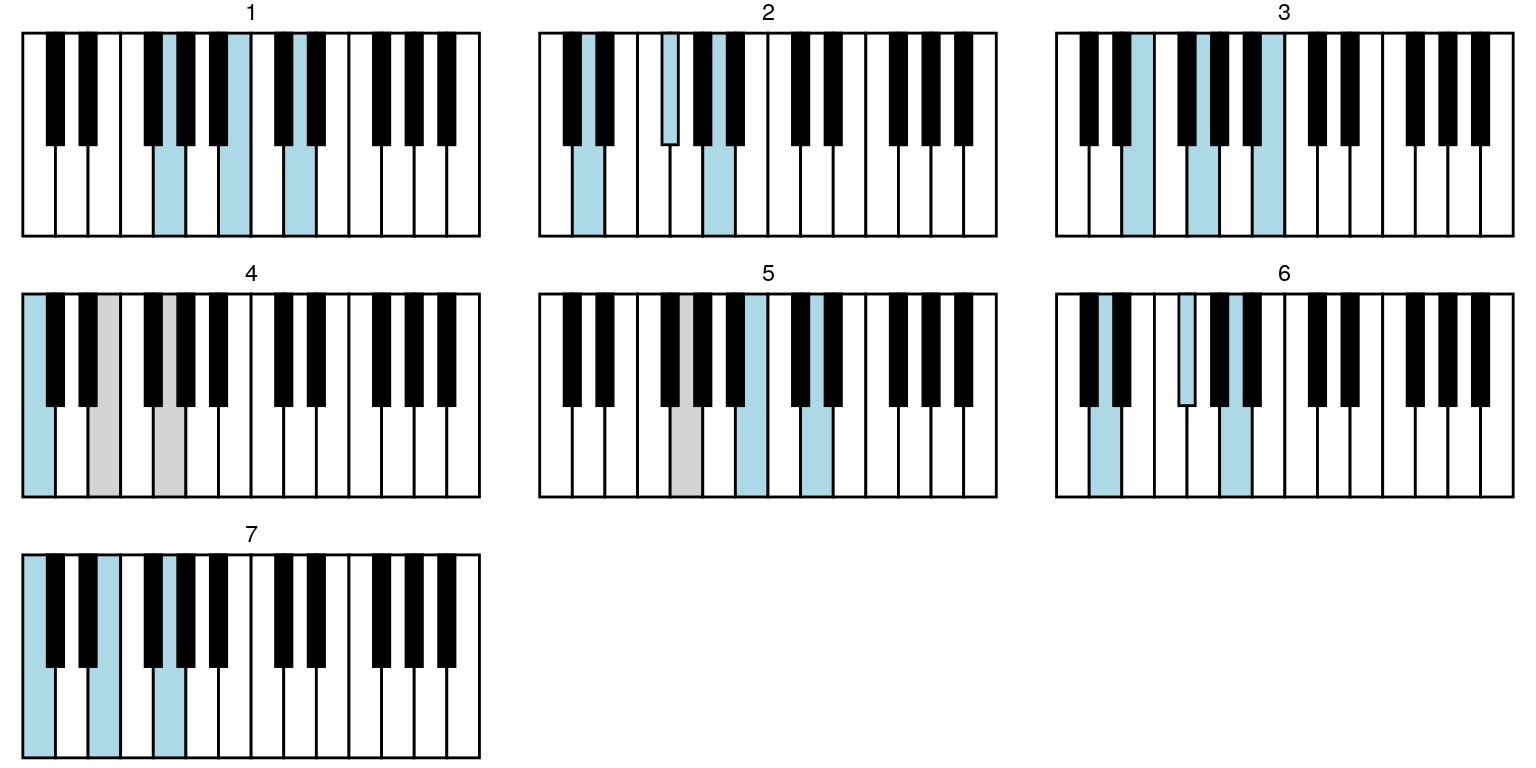And get more helpful facet panel title by providing sequence_names argument to highlight_key_sequence:

keys_chords %>%
highlight_key_sequence(key_sequence = key_seq,
sequence_names = chord_names) %>%
ggpiano() +
facet_wrap(~ seq_name)
#> NULL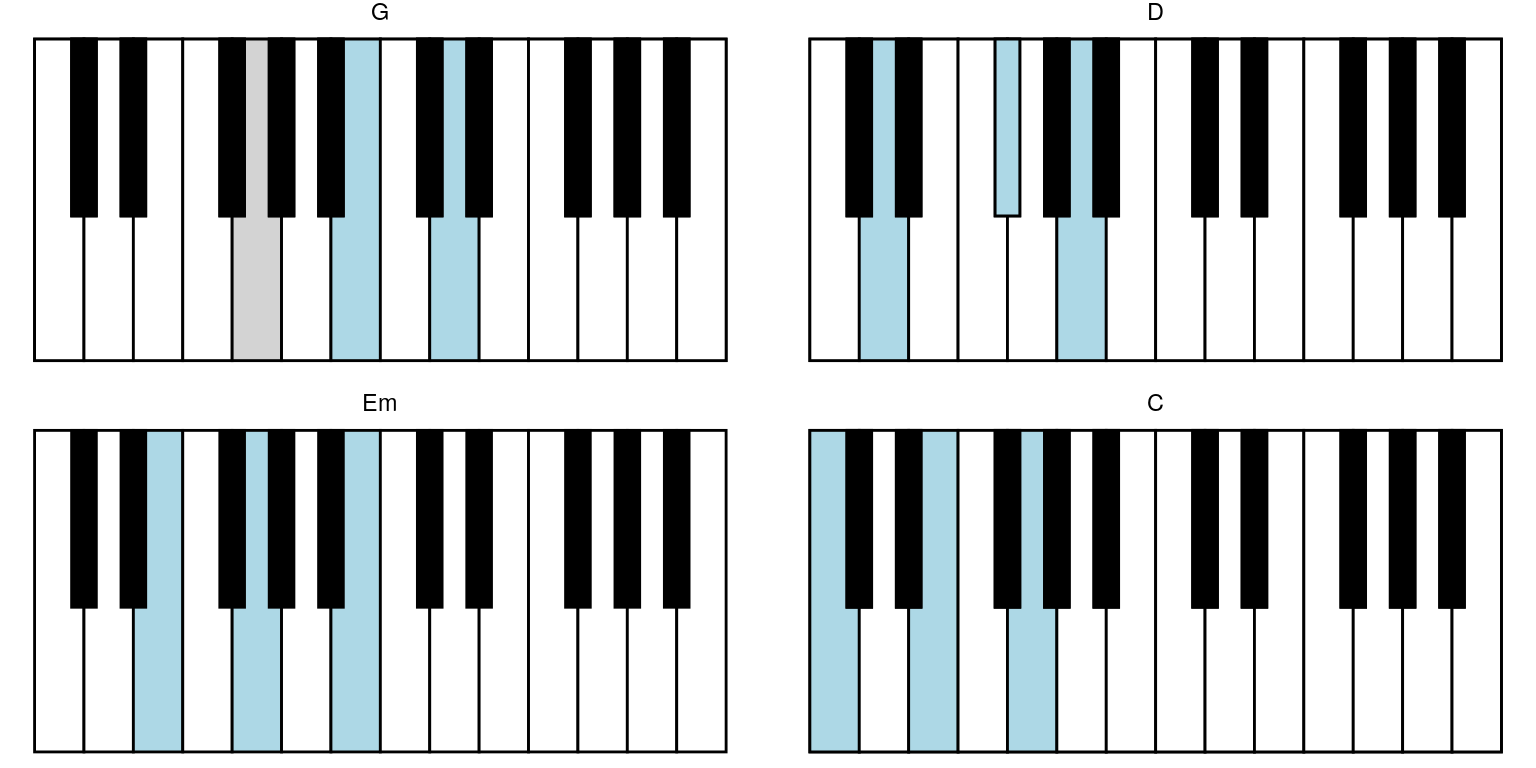And for exmple include seq_no in a new column seq_lbl (that are then converted to a factor with the right ordering):

keys_chords %>%
highlight_key_sequence(key_sequence = key_seq,
sequence_names = chord_names) %>%
mutate(seq_lbl = paste0(seq_name, " (#", seq_no, ")")) %>%
mutate(seq_lbl = forcats::fct_inorder(seq_lbl)) %>%
ggpiano() +
facet_wrap(~ seq_lbl)
#> NULL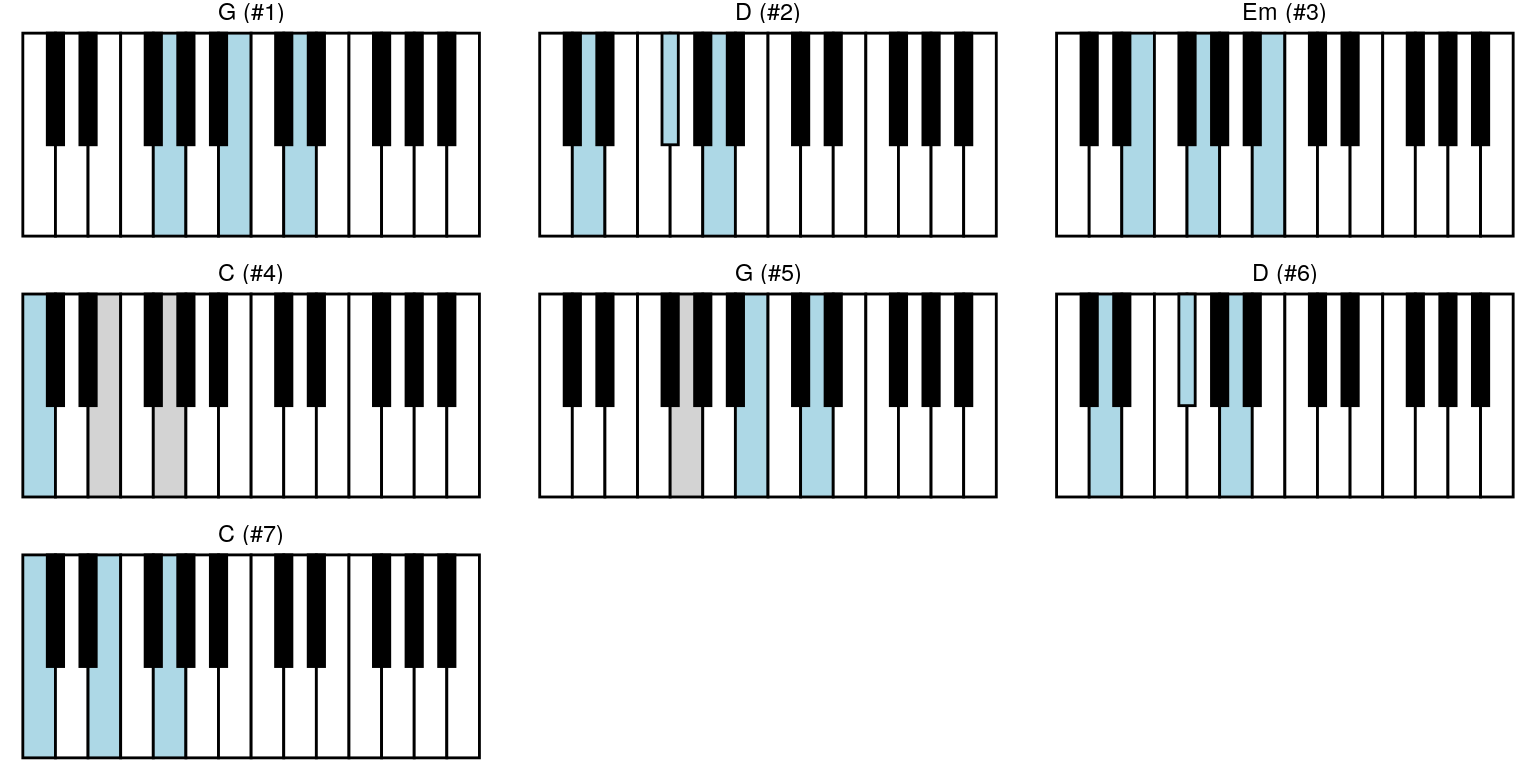# Finding better inversions

Currently only one method is available for finding better inversions. It is minimising the distances between keys in consecutive chords, where distance is the number of keys not used anymore plus new keys.
And with an exhaustive approach that is only feasible for shorter sequences.

opt_res <- optim_min_dist_exhaustive(key_sequence = key_seq)
keys_chords %>%
highlight_key_sequence(key_sequence = opt_res$best, sequence_names = chord_names) %>% ggpiano() + facet_wrap(~ seq_name) + labs(title = "Best inversions") #> NULL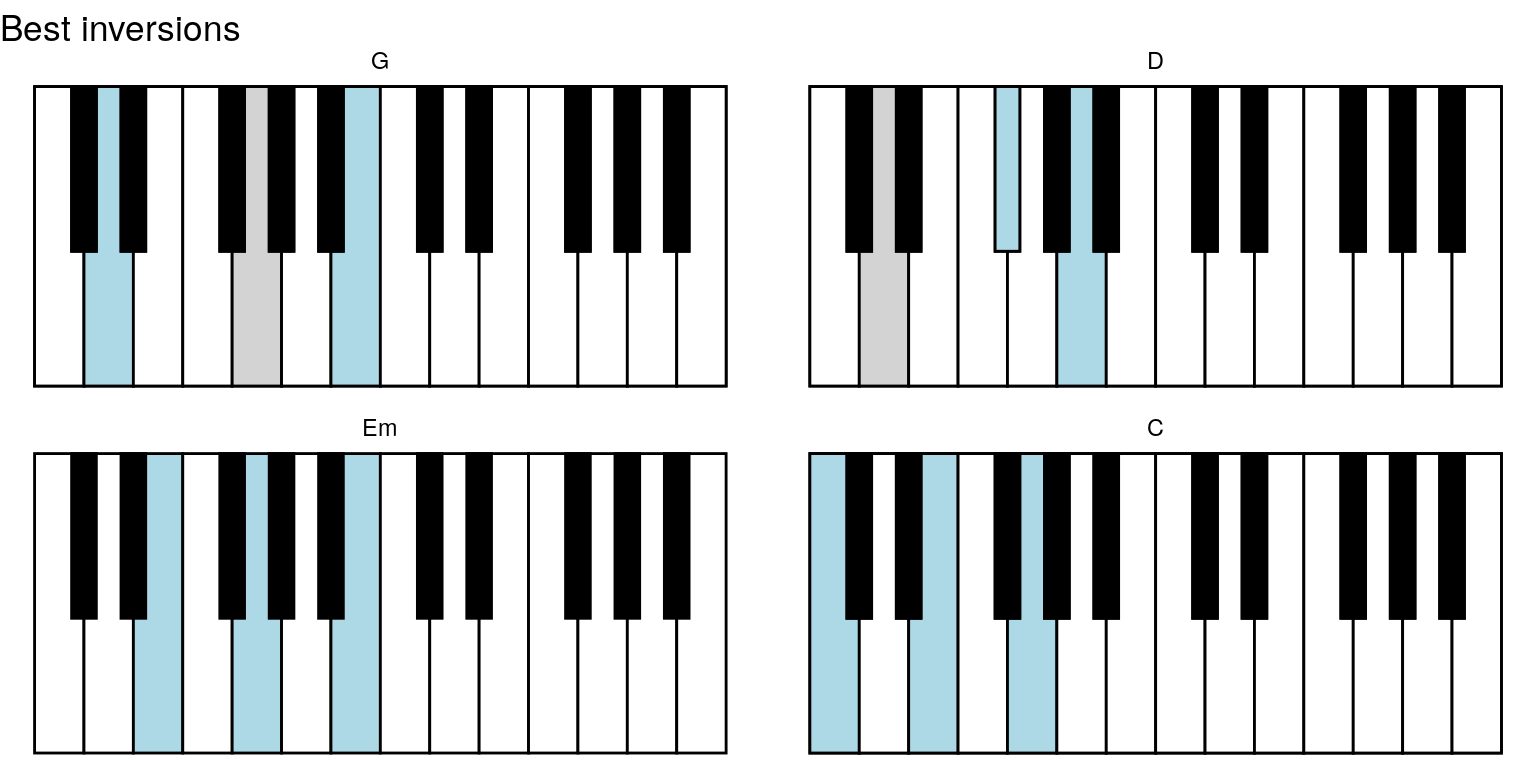keys_chords %>% highlight_key_sequence(key_sequence = opt_res$worst,
sequence_names = chord_names) %>%
ggpiano() +
facet_wrap(~ seq_name) +
labs(title = "Worst inversions")
#> NULL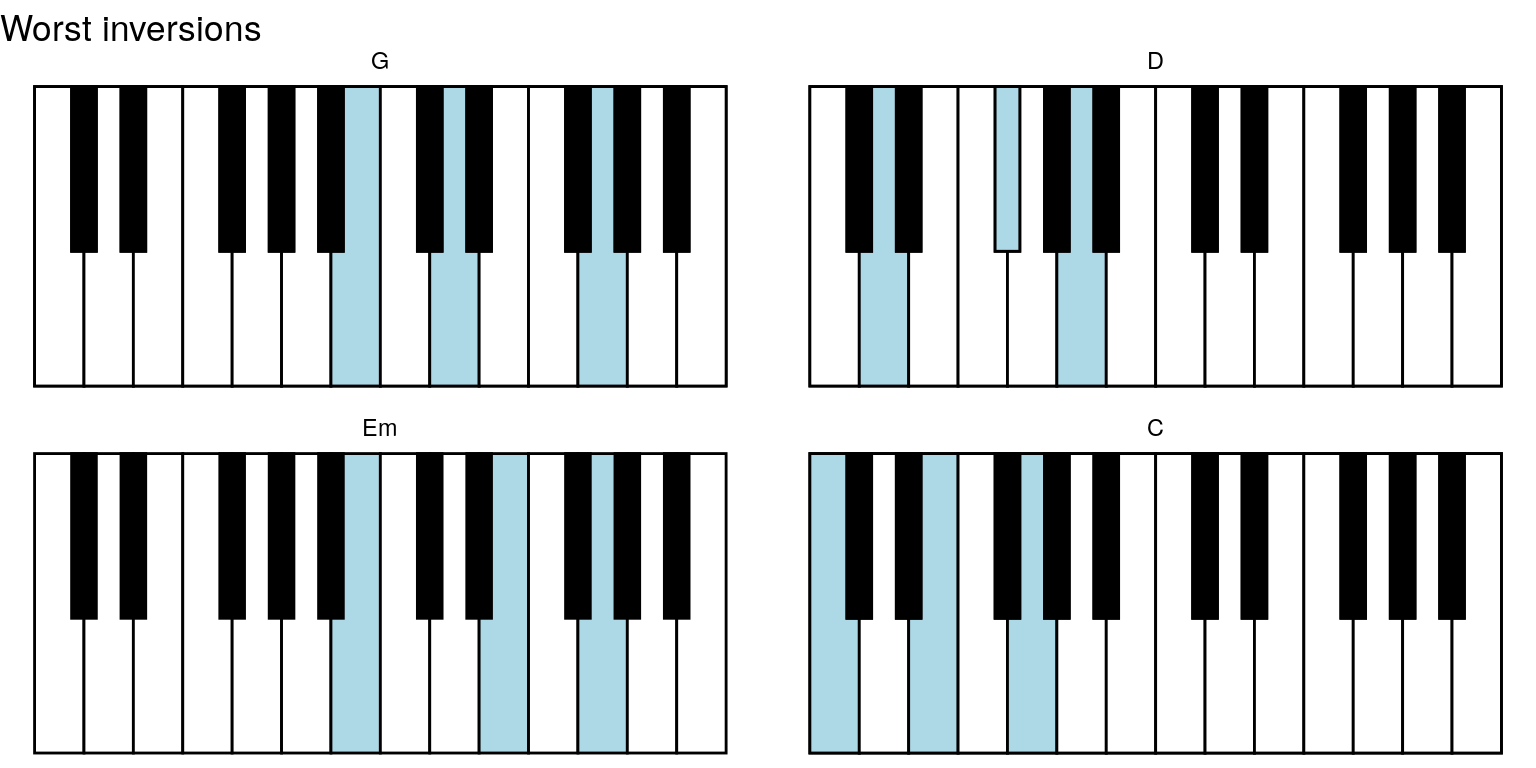# Bigger example

chords <- list(
list(chord = construct_chord_major("G"),
highest_tone = "G"),

list(chord = construct_chord_minor("B"),
highest_tone = "F#"),

list(chord = construct_chord_major("C"),
highest_tone = "E"),

list(chord = construct_chord_major("G"),
highest_tone = "D")
)
chord_keys <- lapply(chords, function(x) get_keys_highest_tone(x$chord, x$highest_tone))
chord_names <- lapply(chords, function(x) paste0(
as.character(x$chord, brief = TRUE), " with ", x$highest_tone, " highest"))

keys_chords %>%
highlight_key_sequence(key_sequence = chord_keys,
sequence_names = chord_names) %>%
ggpiano() +
facet_wrap(~ seq_name)
#> NULL